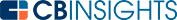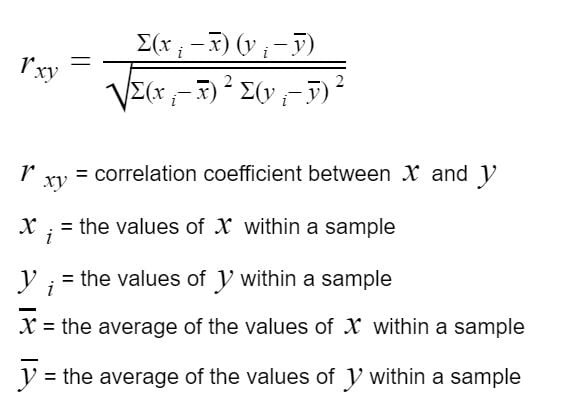# What is the Correlation Formula?In the financial realm, correlation is a measurement of the relationship between two securities. The correlation coefficient is expressed as a number between -1.0 and 1.0.

If the correlation coefficient is -1.0, the two securities are moving in opposite directions. If the number is at 1.0, the two securities move in the same direction. A correlation coefficient of 0 means the two securities have no linear relationship.

## How to calculate the correlation formula

The correlation formula is:It’s recommended that you use a software tool like Microsoft Excel to calculate the correlation coefficient. This is done using the CORREL formula function in Excel.

## How is the correlation coefficient calculated manually?

Let’s look at an example for calculating the correlation coefficient manually.

There are two holdings named Company A and Company B, with data from the previous three years being analyzed.

Step One: First obtain the average price of both companies in the desired timeframe.

The average price of Company A between 2018 and 2020 is \$55.97 [(44.01 + 55.78 + 68.13) / 3) = 55.97].

The average price of Company B between 2018 and 2020 is \$76.93 [(62.87 + 77.02 + 90.89) / 3) = 76.93].Step Two: Next, take each price and subtract its respective average.

Start by taking the price of Company A in 2018 (44.01) and subtracting the average of Company A (55.97) to equal -11.96. Repeat this for the prices of Company A in 2019 and 2020. The results of these equations are in column a.

Next, take the price of Company B in 2018 (62.87) and subtract the average of Company B (76.93) to equal -14.06. Repeat this for the prices of Company B in 2019 and 2020. These results are in column b.Step Three: Then multiply the variables in column a by their respective variables in column b and then calculate the sum of these results.

This is done by multiplying -11.96 (column a) by -14.06 (column b) in the first row to get a result of 168.16 and repeat the process for the next two rows. Finally, add the results together and for the sum at the bottom of the column (337.89).Step Four: Take the values in column a and column b and square them.

Start with column a in 2018 (11.96^2 = 143.04) and repeat for all the values in both columns. Then find the sum of a^2 and b^2 listed at the bottom of the columns.Finally, plug the numbers into the formula below, resulting in a correlation coefficient of 0.9998.This means Company A and Company B have a very high correlation with each other.

## What is the correlation coefficient formula used for?

The correlation coefficient formula has a number of uses when calculating the relationship between two variables. One example would be to compare the price of a commodity with the stock price of a company that produces that commodity. Similarly, the formula could also be used to calculate the correlation between the availability of a raw material (such as steel) and the output of a company that requires that material.

You may also like# www.cbinsights.com